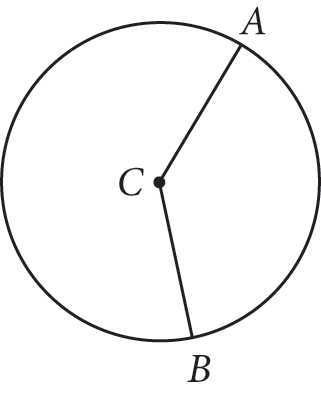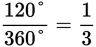# SAT Math Multiple Choice Question 971: Answer and Explanation

### Test Information

Question: 971

7.Points A and B lie on circle C as shown. The measure of angle ACB is 120°. If the area of circle C is 81π square units, what is the length of minor arc AB?

• A. 6π
• B. 9π
• C. 18π
• D. 27π

Getting to the Answer: You know that the area of the circle is 81π. The area of a circle is given by A = πr2, so r = 9. This means the circumference of the circle, which is given by C = 2πr, is C = 2π(9) = 18π. Now, find the portion of the circumference that minor arc AB represents by writing the measure of the central angle that subtends the arc over 360° (the number of degrees in a full circle):. The arc represents one-third of the circumference, or 18π ÷ 3 = 6π, which is (A).